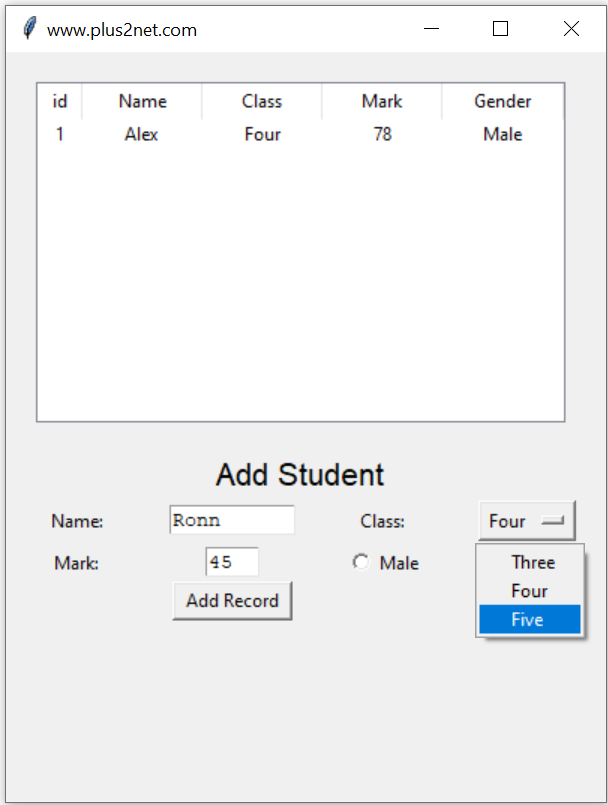# Adding rows using insert() in Tkinter TreeviewWe can use insert() to add one parent of main node ( row ) to the Treeview. Here we have one student record table ( no database here ) and one record is already available. Below the Treeview, input boxes and options are available to add nodes to this Treeview using insert() method.

## Declaring a Treeview

Here we have declared one Treeview and use the object trv for further management of the Treeview.
``````trv=ttk.Treeview(my_w,selectmode='browse')

``insert(parent, index, iid=None, **kw)``
`parent` : For Parent row it should be '', for child row parent iid to be used.
`index` : Index position of row. To added at the bottom 'end' and 0 if at top.
`iid` : Optional, Identifier of the item ( row ), Treeview will create unique iid if not given.
`text` : Text to appear at right of icon column.

Using this after adding header and columns ( check the code below ) one record is added with iid=1 ( unique id of the row )
``````i=1
trv.insert("",'end',iid=i,
values=(i,'Alex','Four',78,'Male'))``````
Below this Treeview we will provide inputs using Label, Text, OptionMenu and Radio buttons to take one input row data and add to the row on Click of a Button.
``````b1 = tk.Button(my_w,  text='Add Record', width=10,
b1.grid(row=6,column=2)``````
The function add_data() will be executed once the Button b1 is clicked. Inside this function we will read the user entered data and then add the row to our Treeview. Here we are not validating any input data.
``````def add_data():

global i
i=i+1
trv.insert("",'end',values=(i,my_name,my_class,my_mark,my_gender))
t1.delete('1.0',END)  # reset the text entry box
t3.delete('1.0',END)  # reset the text entry box
t1.focus()
l5.after(3000, lambda: my_str.set('') ) # remove the message   ``````
To add the row at the top change this line
``trv.insert("",0,values=(i,my_name,my_class,my_mark,my_gender))``

Adding data to Tkinter Treeview using insert() to update view & place the data in top or bottom row

The full code with layout of the window is here.
``````from tkinter import ttk
import tkinter as tk
from tkinter import *
my_w=tk.Tk()
my_w.geometry('400x500')
my_w.title("www.plus2net.com")
trv=ttk.Treeview(my_w,selectmode='browse')
trv["columns"]=("1","2","3","4","5")
trv.column("1",width=30,anchor='c')
trv.column("2",width=80,anchor='c')
trv.column("3",width=80,anchor='c')
trv.column("4",width=80,anchor='c')
trv.column("5",width=80,anchor='c')
i=1
trv.insert("",'end',iid=i,
values=(i,'Alex','Four',78,'Male'))

font=('Helvetica', 16), width=30,anchor="c" )
l0.grid(row=2,column=1,columnspan=4)

l1 = tk.Label(my_w,  text='Name: ', width=10,anchor="c" )
l1.grid(row=3,column=1)

t1 = tk.Text(my_w,  height=1, width=10,bg='white')
t1.grid(row=3,column=2)

l2 = tk.Label(my_w,  text='Class: ', width=10 )
l2.grid(row=3,column=3)

# add list box for selection of class
options = StringVar(my_w)
options.set("") # default value

opt1 = OptionMenu(my_w, options, "Three", "Four", "Five")
opt1.grid(row=3,column=4)

l3 = tk.Label(my_w,  text='Mark: ', width=10 )
l3.grid(row=5,column=1)

t3 = tk.Text(my_w,  height=1, width=4,bg='white')
t3.grid(row=5,column=2)

r1.grid(row=5,column=3)

r2.grid(row=5,column=4)

b1 = tk.Button(my_w,  text='Add Record', width=10,
b1.grid(row=6,column=2)
my_str = tk.StringVar()
l5 = tk.Label(my_w,  textvariable=my_str, width=10 )
l5.grid(row=8,column=1)

global i
i=i+1
trv.insert("",'end',
values=(i,my_name,my_class,my_mark,my_gender))
t1.delete('1.0',END)  # reset the text entry box
t3.delete('1.0',END)  # reset the text entry box
t1.focus()
l5.after(3000, lambda: my_str.set('') ) # remove the message
my_w.mainloop()``````

## Using Database

We can add rows to Treeview only after getting confirmation of adding record to MySQL database. This we will add next.

Subscribe to our YouTube Channel here

## Subscribe

* indicates required
Subscribe to plus2netplus2net.com